Anatomy Of Mean Reversion in EasyLanguage

Look at this equity curve: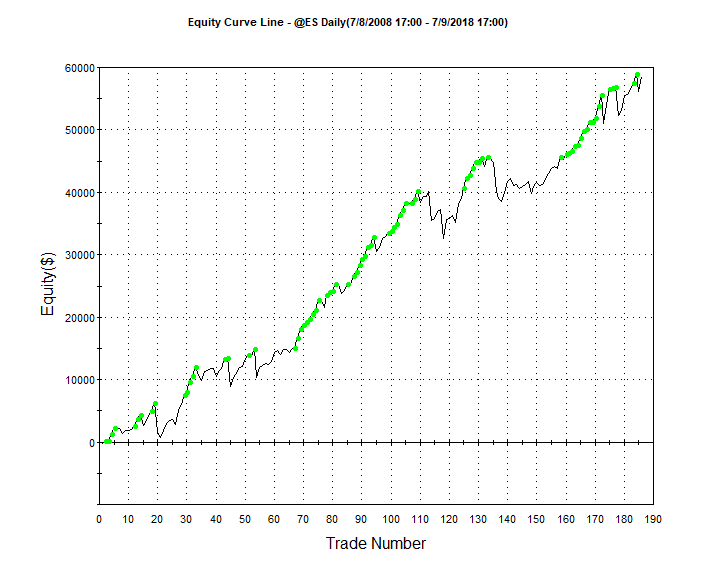As long as you are in a bull market buying dips can be very consistent and profitable.  But you want to use some type of entry signal and trade management other than just buying a dip and selling a rally.  Here is the anatomy of a mean reversion trading algorithm that might introduce some code that you aren’t familiar.  Scroll through the code and I will  summarize below.

``````inputs: mavlen(200),rsiLen(2),rsiBuyVal(20),rsiSellVal(80),holdPeriod(5),stopLoss\$(4500);

Condition1 = c > average(c,mavLen);

Condition3 = rsi(c,rsiLen) > rsiSellVal;

Value1 = 0;
Value2 = 0;

For iCnt = 0 to consecUpClose - 1
Begin
value1 = value1 + iff(c[iCnt] > c[iCnt+1],1,0);
end;

For iCnt = 0 to consecDnClose - 1
Begin
Value2 = value2 + iff(c[iCnt] < c[iCnt+1],1,0);
end;

dontCatchFallingKnife = absValue(C - c) < avgTrueRange(10)*2.0;

meanRevBuy = condition1 and condition2 and dontCatchFallingKnife;
meanRevSell =  not(condition1) and condition3 and dontCatchFallingKnife;

If marketPosition = 1 and condition1 and value1 >= consecUpClose then sell("ConsecUpCls") this bar on close;

If meanRevSell then sellShort this bar on close;
If marketPosition = -1 and not(condition1) and value2 >= consecDnClose then buyToCover this bar close;

setStopLoss(stopLoss\$);

Begin
if marketPosition = 1 and not(meanRevBuy) then sell this bar on close;
if marketPosition =-1 and not(meanRevSell) then buytocover this bar on close;
end;``````
Mean Reversion System

I am using a very short term RSI indicator, a la Connors, to initiate long trades.  Basically when the 2 period RSI dips below 30 and the close is above the 200-day moving average I will buy only if I am not buying “a falling knife.”  In February several Mean Reversion algos kept buying as the market fell and eventually got stopped out with large losses.  Had they held on they probably would have been OK.  Here I don’t buy if the absolute price difference between today’s close and yesterday’s is greater than 2 X the ten day average true range.  Stay away from too much “VOL.”

Once a trade is put on I use the following logic to keep track of consecutive closing relationships:

``````For iCnt = 0 to consecUpClose - 1
Begin
value1 = value1 + iff(c[iCnt] > c[iCnt+1],1,0);
end;``````
Using the IFF function in EasyLanguage

Here I am using the IFF function to compare today’s close with the prior day’s.  iCnt is a loop counter that goes from 0 to 1. IFF checks the comparison and if it’s true it returns the first value after the comparison and if false it returns the last value.  Here if I have two consecutive up closes value1 accumulates to 2.  If I am long and I have two up closes I get out.  With this template you can easily change this by modifying the input:  consecUpClose.  Trade management also includes a protective stop and a time based exit.  If six days transpire without two up closes then the system gets out – if the market can’t muster two positive closes, then its probably not going to go anywhere.  The thing with mean reversion, more so with other types of systems, is the use or non use of a protective stop.  Wide stops are really best, because you are betting on the market to revert.  Look at the discrepancy of results using different stop levels on this system: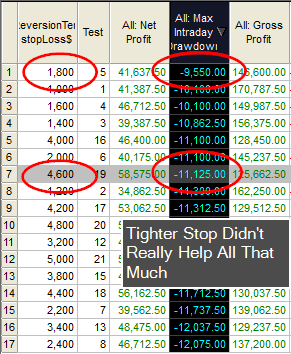Here an \$1,800 stop only cut the max draw down by \$1,575.  But it came at a cost of \$17K in profit.  Stops, in the case of Mean Reversion, are really used for the comfort of the trader.

This code has the major components necessary to create a complete trading system.  Play around with the code and see if you can come up with a better entry mechanism.

Update To Original Pattern Smasher

What will you learn : string manipulation, for-loops, optimization

Before proceeding I would suggest reading my original post on this subject.    If you believe the relationship of the last few bars of data can help determine future market direction, then this post will be in you wheel house.  Another added benefit is that you will also learn some cool EasyLanguage.

Original post was limited to four day patterns!

This version is limitless (well not really, but pretty close).  Let’s stick with the original string pattern nomenclature (+ + – – : two up closes followed by two down closes.)  Let’s also stick with our binary pattern representation:

 Pattern # 2^3 2^2 2^1 1 3 0 0 1 1 4 0 1 0 0 5 0 1 0 1 6 0 1 1 1

Remember a 0 represents a down close and a 1 represents an up close.  We will deviate from the original post by doing away with the array and stick with only strings (which are really just arrays of characters.)  This way we won’t have to worry about array manipulation.

How to create a dynamic length string pattern

This was the difficult part of the programming.  I wanted to be able to optimize 3, 4 and 5 day patterns and I wanted to control this with using just inputs.  I discovered that pattern three is different in a three day pattern than it is in a four day pattern: in a three day pattern it is 011 or – + + and in a four day pattern it is 0011 or – – + +.  Since I am counting 0’s as down closes, pattern #3 depends on the ultimate size of the pattern string.  No worries I will have eventually have another version where I utilize a different value for down closes and we can then have holes in our string patterns.  But I digress – so to differentiate the patterns based on the pattern length I included a maxPatternLen input.  So if maxPatternLen is three and we are trying to match pattern #3 then we will be looking for 011 and not 0011.  That was an easy fix.  But then I wanted to build a string pattern based on this input and the pattern number dynamically.  Here is some psuedo code on how I figured it out.

``````
{Psuedo code to translate pattern number into binary number}
patternNumber = 3
maxPatternLen = 3

numBits = 0    						// stick with binary representation
testValue = 0						// temporary test value
numBits = maxPatternLen-1  			// how many bits will it take to get to the
// center of - or numBits to represent max
// number of patterns or 2^numBits
currentBit =numBits					// start wit current bit as total numBits

value1 = patternOptTest				// value1 represents current pattern number
testString = ""  					// build test string from ground up

for icnt = numBits downto 0			//building string from left to right
begin       						// notice keyword downto
if power(2,currentBit) > value1 then  // must use power function in EL
begin							// if the very far left bit value >
testString = testString + "-"	  // patten number then plug in a "-"
end
else
begin							// else plug in a "+" and deccrement by
testString = testString + "+"	 // that bits value - if its the 3rd bit
value1 = value1 - power(2,currentBit)// then decrement by 8
end;
currentBit = currentBit - 1		// move onto the next bit to the right
end;``````
Pseudocode for Binary Representation of Pattern #

Now if you want to optimize then you must make sure your pattern number search space or range can be contained within maxPatternLen.  For example, if you want to test all the different combinations of a four day pattern, then your maxPatternLen would naturally be four and you would optimize the pattern number from 0 to 15.  Don’t use 1-16 as I use zero as the base.  A five day pattern would include the search space from 0 – 31.  The rest of the code was basically hacked from my original post.   Here is the rest of the code to do optimizations on different length pattern strings.  Notice how I use strings, for-loops and comparisons.

``````input: buyPattern("+++-"),sellPattern("---+"),patternOptimize(True),patternOptTest(7),maxPatternLen(3),patternOptBuySell(1),
stopLoss\$(2000),profitTarg\$(2000),holdDays(5);

sellPatternMatch = sellPattern;
numCharsInSellPattern = strLen(sellPatternMatch);

If patternOptimize then
begin
numBits = 0;
testValue = 0;
value = maxPatternLen;
numBits = maxPatternLen-1;
currentBit =numBits;
remainder = patternOptTest;
testString = "";
for icnt = numBits downto 0
begin
if power(2,currentBit) > value1 then
begin
testString = testString + "-";
end
else
begin
testString = testString + "+";
remainder = remainder - power(2,currentBit);
end;
currentBit = currentBit - 1;
end;
numCharsInSellPattern = maxPatternLen;
Begin
sellPatternMatch = "0";
end;
Begin
sellPatternMatch = testString;
end;
end;

sellPatternString = "";

For icnt = numCharsInBuyPattern-1 downto 0
Begin
end;
For icnt = numCharsInSellPattern-1 downto 0
Begin
If close[icnt] >= close[icnt+1] then sellPatternString = sellPatternString + "+";
If close[icnt] < close[icnt+1] then sellPatternString = sellPatternString + "-";
end;

okToSell = false;

If sellPattern <> "" then
If sellPatternString = sellPatternMatch then okToSell = true;
If sellPatternMatch = "" then
okToSell = true;

If okToSell then sellshort next bar at open;

If marketPosition = 1 and barsSinceEntry > holdDays then sell next bar at open;
If marketPosition = -1 and barsSinceEntry > holdDays then buytocover next bar at open;

setStopLoss(stopLoss\$);
setProfitTarget(profitTarg\$);

Final Version of New Pattern Smasher

Also see how I incorporate a profit target and protective stop.  I use the built in BarsSinceEntry function to count the number of days I am in a trade so I can utilize a time based exit.  Here is an interesting equity curve I developed using a two day pattern ( – –) to go long.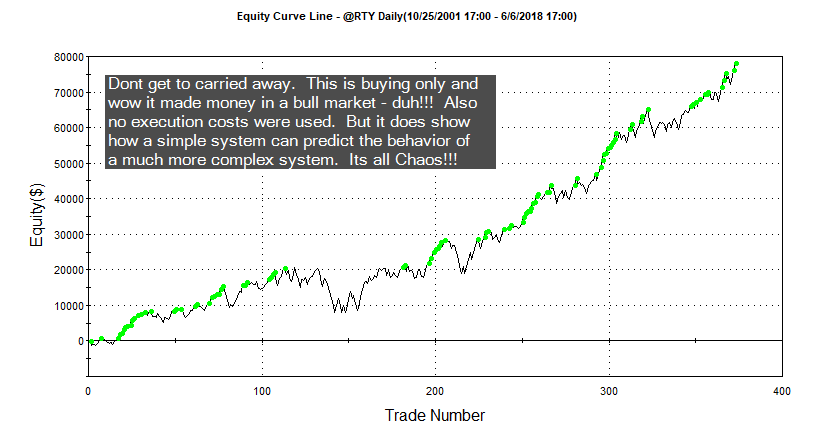Register on the website and I will email you an ELD of the improved Pattern Smasher.  Or just shoot me an email.

Pyramiding and then Scaling Out at Different Price Levels – EasyLanguage

TOTAL, TOTAL, TOTAL – an important keyword

I just learned something new!  I guess I never programmed a strategy that pyramided at different price levels and scaled out at different price levels.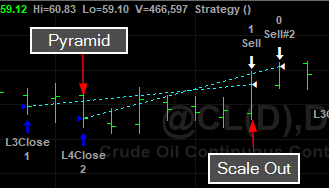Initially I thought no problem.  But I couldn’t get it to work – I tried everything and then I came across the keyword Total and then I remembered.  If you don’t specify Total in you exit directives then the entire position is liquidated.  Unless you are putting all your positions on at one time – like I did in my last post.   So remember if you are scaling out of a pyramid position use Total in your logic.

``````vars: maxPosSize(2);

If currentContracts < maxPosSize - 1 and c > average(c,50) and c = lowest(c,3) then buy("L3Close") 1 contract this bar on close;
If currentContracts < maxPosSize and c > average(c,50) and c = lowest(c,4) then buy("L4Close") 1 contract this bar on close;

If currentContracts = 2 and c = highest(c,5) then sell 1 contract total this bar on close;
If currentContracts = 1 and c = highest(c,10) then sell 1 contract total this bar on close;``````
Scaling Out Of Pyramid

Why you have to use the Total I don’t know.  You specify the number of contracts in the directive and that is sufficient if you aren’t pyramiding.  The pyramiding throws a “monkey wrench” in to the works.

First Put Multiple Contracts On:

``If c > average(c,200) and c = lowest(c,3) then buy("5Large") 5 contracts this bar on close;``
Using keyword contracts to put on multiple positions

Here you specify the number of contracts prior to the keyword contracts.

Easylanguage requires you to create a separate order for each exit.  Let’s say you want to get out of the 5 positions at different times and possibly prices.  Here’s how you do it:

``````If currentContracts = 5 and c > c then sell 1 contracts this bar on close;
If currentContracts = 4 and c > c then sell 1 contracts this bar on close;
If currentContracts = 3 and c > c then sell 1 contracts this bar on close;
If currentContracts = 2 and c > c then sell 1 contracts this bar on close;
If currentContracts = 1 and c > c then sell 1 contracts this bar on close;``````
One order for each independent exit

The reserved word currentContracts hold the current position size.  Intuitively this should work but it doesn’t.

{If currentContracts > 0 then sell 1 contract this bar on close;}

You also can’t put order directives in loops.  You can scale out using percentages if you like.

``````Value1 = 5;

If currentContracts = 5 and c > c then sell 0.2 * Value1 contracts this bar on close;
If currentContracts = 4 and c > c then sell 1 contracts this bar on close;
If currentContracts = 3 and c > c then sell 1 contracts this bar on close;
If currentContracts = 2 and c > c then sell 1 contracts this bar on close;
If currentContracts = 1 and c > c then sell 1 contracts this bar on close;``````
Using a percentage of original order size

That’s all there is to scaling out.  Just remember to have an independent exit order for each position you are liquidating.  You could have just two orders:  scale out of 3 and then just 2.

Setting Stop Loss and Profit Target Utilizing EntryPrice with EasyLanguage

One Problem with the “Next Bar” Paradigm – market position nor entryPrice are adjusted by the end of the bar

Whenever I develop a strategy I like to program all of my entries and exits without utilizing TradeStations built-in execution functions.  I just got use to doing this when I started programming in Fortran many years ago.  However, there a few scenarios where this isn’t possible.  If you enter a trade and use the following logic to get you out with a loss or a profit when referencing your entryPrice, you will be surprised with your results.  This is because you are telling the computer to use entryPrice before you know what it is.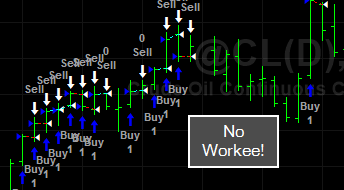This logic is absolutely correct in its intention.  However, TradeStation doesn’t realize you are in a position at the end of the bar and can’t properly reference entryPrice.  Okay so we force TradeStation to only issue orders once it has a valid entryPrice.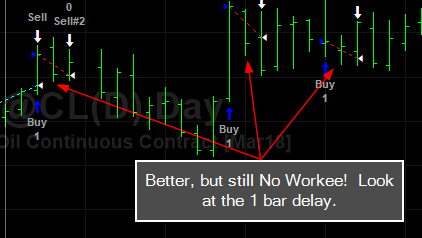TradeStation only realizes the correct marketPosition the following day and then issues an order for the next bar.  So we get the one bar delay.  It would be helpful if TradeStation would set the marketPosition at the close of the bar on the bar of entry.   However, you can overcome this with TradeStation’s built-in execution functions.  For some reason these functions know exactly when you get in – you can also get the same results by inserting the respective strategies on the chart.

An Easy Fix ThoughBut this little bug can creep into other areas of your programming.  Keep an eye on this.

Re-Entry After Taking A Profit

Here is some code I have been working on.  I will go into detail on the code a little later.  But this is how you monitor re-entering at a better price after taking a profit.  The problem with taking profits on longer term trend following systems is that the logic that got you into the position is probably still true and you will notice your algorithm will re-enter in the same direction.  So you need to inform your algorithm not to re-enter until a certain condition is met.  In this example, I only re-enter at a better price if the condition that got me into the trade is still valid.

``````Inputs: swingHiStrength(2),swingLowStrength(2),numDaysToLookBack(30),stopAmt\$(500),profitAmt\$(1000),getBackInAfterProfAmt\$(250);
vars: mp(0),longProfTaken(false),shortProfTaken(false);

mp = marketPosition;

if not(longProfTaken) and mp <> 1 then Buy("BreakOut-B") next bar at highest(h,20) on a stop;
if not(shortProfTaken) and mp <>-1 then SellShort("BreakOut-S") next bar at lowest(l,20)  on a stop;

//If mp = 0 and mp = 1 then print(date," ",exitPrice(1)," ",entryPrice(1));

If longProfTaken then
Begin
If c < exitPrice(1) - getBackInAfterProfAmt\$/bigPointValue then
begin
longProfTaken = false;
If mp <> -1 then buy("longRe-Entry") next bar at open;
end;
end;

If shortProfTaken then
Begin
If c > exitPrice(1) + getBackInAfterProfAmt\$/bigPointValue then
begin
shortProfTaken = false;
If mp <>-1 then sellShort("shortRe-Entry") next bar at open;
end;
end;

If mp = 1 and c > entryPrice + profitAmt\$/bigPointValue then
begin
sell this bar on close;
longProfTaken = true;
end;

If mp =-1 and c < entryPrice - profitAmt\$/bigPointValue then
begin
shortProfTaken = true;
end;

If mp = -1 then longProfTaken = false;
If mp = 1 then shortProfTaken = false;

//if mp = 1 then setStopLoss(stopAmt\$);
//if mp = 1 then setProfitTarget(profitAmt\$);``````
Re-Entering At Better Price After Profit

A Slightly More Eloquent Approach to Programming Our Pyramiding E-Mini DayTrading Algorithm.

Okay let’s see how I was able to add some eloquence to the brute force approach to this pyramiding algorithm.  The original code included multiple entry directives and a ton of hard coded numerical values.   So let me show you how I was able to refine the logic/code and in doing so make it much more flexible.  We might lose a little bit of the readability, but we can compensate by using extra commentary.

First off, let’s add flexibility by employing input variables.  In this case, we need to inform the algorithm the distance from the open to add additional positions and the max number of entries allowed for the day.

``inputs : pyramidDistance(5),maxDailyEntries(3);``

Now we need to set somethings up for the first bar of the day.  Comparing the date of today with the date of yesterday is a good way to do this.

``````if d<>d then
begin
canSell = true;
sellMult = 1;
sellStop = -999999;
entries = 0;
end;``````
First bar of the day housekeeping.

Here is a neat way to keep track of the number of entries as they occur throughout the trading day.  Remember the function EntriesToday(date) will not provide the information we need.

``````mp = marketPosition * currentShares;

if mp <> mp and mp <> 0 then entries = entries + 1;``````
How to track the number of entries for today.

If the last bar’s mp is not equal to the current bar’s mp then and mp is not equal to zero then we know we have added on another entry.  Okay now let’s think about eliminating the “brute force” approach.

Instead of placing multiple order entry directives I  only want to use one with a variable stop level.  This stop level will be guided by the variable SellMult.  We start the day with a wacky sell stop level and then calculate it based on the SellMult variable and PyramidDistance input.

``````if low <= sellStop  then
begin
sellMult = sellMult + 1;
end;

sellStop = openD(0) - sellMult * pyramidDistance;``````
Calculate and adapt sell stop level as we go along.

So on the first bar of the day the sellStop = openD(0) – sellMult * pyramidDistance or sellStop = openD(0) – 1 * 5.  Or 5 handles below the open.  Note you an change the pyramidDistance input and make it three to match the previous examples.

``````if entries = maxDailyEntries then canSell = false;
if time < sess1EndTime and canSell then sellShort 1 contract next bar at sellStop stop;
if mp <=-1 {and barsSinceEntry > 0} then buyToCover next bar at sellStop + 2* pyramidDistance stop;

setexitonclose;``````
That's it! Pretty simple isn't it?

Ok, we need to tell the computer to turn off the ability to place orders if one of two things happens:  1) we have reached the maxDailyEntries or 2) time >= sess1EndTime.    You could make the time to stop entering trades an input as well.  If neither criteria applies then place an order to sellShort at our sellStop level.   If price goes below our sell stop level then we know we have been filled and the new sellStop level needs to be recalculated.  See how we use a calculation to adapt the stop level with a single order placement directive?  This is where the eloquence comes into play.  QED.

Now you code the opposite side and then see if you can make money  (hypothetically speaking of course) with it.  If you think about it, why does this not work.  And the not so obvious reason is that it trades too much.  Other than trading too much it makes perfect sense – buy or sell by taking a nibbles at the market.  If the market takes off then take a big bite.  The execution costs of the nibbles are just way too great.  So we need to think of a filtering process to determine when it is either better to buy or sell or when to trade at all.  Good Luck with this ES [emini S&P ]day trading algorithm!

``````inputs : pyramidDistance(5),maxDailyEntries(3);
vars: mp(0),icnt(0),sellStop(0),sellMult(0),canSell(true),entries(0);

if d<>d then
begin
canSell = true;
sellMult = 1;
sellStop = -999999;
entries = 0;
end;

mp = marketPosition * currentShares;

if mp <> mp and mp <> 0 then entries = entries + 1;
if mp = -1 and mp = 0 then canSell = false;
if time > 1430 then canSell = false;

if low <= sellStop  then
begin
sellMult = sellMult + 1;
end;

sellStop = openD(0) - sellMult * pyramidDistance;
if entries = maxDailyEntries then canSell = false;
if time < sess1EndTime and canSell then sellShort 1 contract next bar at sellStop stop;
if mp <=-1 {and barsSinceEntry > 0} then buyToCover next bar at sellStop + 2* pyramidDistance stop;

setexitonclose;``````
Much More Flexible Code

Long Entry Logic and EasyLanguage Code for Pyramiding Algorithm

Sorry for the delay in getting this up on the web.  Here is the flip side of the pyramiding day trade scheme from the buy side perspective.  I simply flipped the rules.  In some cases, to keep the programming a little cleaner I like to keep the buy and sellShort logic in two separate strategies.  So with this chart make sure you insert both strategies.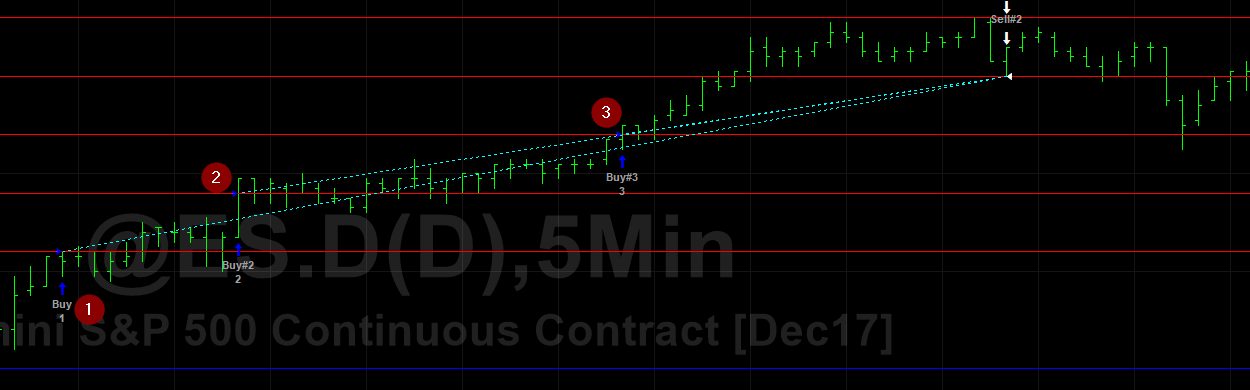And here is the code:

``````vars: mp(0),lastTradePrice(0),canBuy(true);

mp = marketPosition * currentContracts;

if date <> date then
begin
end;

if mp = 1 then canBuy = false;
if time > 1430 then canBuy = false;

if mp = 0 and canBuy = true then buy next bar at OpenD(0) + 3 stop;
if mp = 1 then buy next bar at OpenD(0) + 6 stop;
if mp = 2 then buy next bar at OpenD(0) + 9 stop;

if mp = 1 then lastTradePrice = OpenD(0) + 3;
if mp = 2 then lastTradePrice = OpenD(0) + 6;
if mp = 3 then lastTradePrice = OpenD(0) + 9;

if mp <> 0 then sell next bar at lastTradePrice - 3 stop;

if mp = 3 and barsSinceEntry > 0 and highD(0) > lastTradePrice + 3 then sell next bar at highD(0) - 3 stop;

setExitOnClose;``````
Pyramiding 3 Handles Up and Trailing Stop

Check out the latest video on Pyramiding.

Here is the finalized tutorial on building the pyramiding ES-day-trade system that was presented in the last post.

I will admit this video should be half as long as the end result.  I get a bit long-winded.  However, I think there are some good pointers that should save you some time when programming a similar system.

EasyLanguage Source:

Here is the final code from the video:

``````vars: mp(0),lastTradePrice(0),canSell(true);

mp = marketPosition * currentContracts;

if date <> date then
begin
canSell = true;  // canSell on every day
end;

if mp = -1 then canSell = false; // one trade on - no more
if time > 1430 then canSell = false; //no entries afte 230 central

if mp = 0 and canSell = true then sellShort next bar at OpenD(0) - 3 stop;

if mp = -1 then sellShort next bar at OpenD(0) - 6 stop; //add 1
if mp = -2 then sellShort next bar at OpenD(0) - 9 stop; //add 2

if mp = -1 then lastTradePrice = OpenD(0) - 3; //keep track of entryPrice
if mp = -2 then lastTradePrice = OpenD(0) - 6;
if mp = -3 then lastTradePrice = OpenD(0) - 9;

if mp <> 0 then buyToCover next bar at lastTradePrice + 3 stop; // 3 handle risk on last trade

// next line provides a threshold prior to engaging trailing stop
if mp = -3 and barsSinceEntry > 0 and lowD(0) < lastTradePrice - 3 then buyToCover next bar at lowD(0) + 3 stop;

setExitOnClose;``````
EasyLanguage for Pyramiding and Day-Trading ES

What we learned here:

• can’t use entriesToday(date) to determine last entry price
• must use logic to not issue an order to execute on the first bar of the next day
• mp = marketPosition * currentContracts is powerful stuff!

In the next few days, I will publish the long side version of this code and also a more eloquent approach to the programming that will allow for future modifications and flexibility.

Let me know how it works out for you.

Take this code and add some filters to prevent trading every day or a filter to only allow long entries!

Learn to Program Pyramiding Algorithm

Would you like to learn how to do this?  Check back over the next few days and I will show you to do it.  Warning:  its not straightforward as it seems – some tricks are involved.  Remember to sign up for email notifications of new posts.

UPDATE:  I have recorded an introductory webcast on how to program this pyramiding scheme.  This webcast is Part 1 and illustrates how to brainstorm and start thinking/programming about a problem.  Part 1 introduces some concepts that show how you can use and adapt some of EasyLanguage built-in reserved words and functions.  I start from the perspective of a somewhat beginning EasyLanguage programmer  – one that knows enough to maybe not get the problem solved, but at least get the ball rolling.  The final code may not look anything like the code I present in Part 1.  However it is sometimes important to go down the wrong trail so that you can learn the limitations of a programming language.  Once you know the limitations, you can go about programming workarounds and fixes.  I hope you enjoy Part 1  I should have Part 2 up soon.  Don’t be too critical, this is really the first webcast I have recorded.  You’ll notice I repeat myself and I refer  to one function input as a subscript.  Check it out:  https://youtu.be/ip-DyyKpOTo